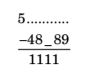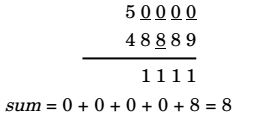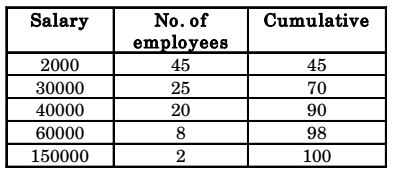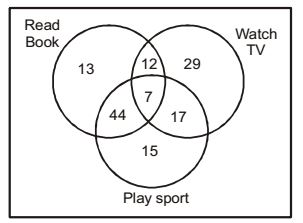## Numerical Ability

#### Numerical Ability

1. What is the sum of the missing digits in the subtraction problem below?1.##### Correct Option: D1. In a company with 100 employees, 45 earn Rs. 20000 per month, 25 earn Rs. 30000,20 earn Rs. 40000,8 earn Rs. 60000, and 2 earn Rs. 150000. The median of the salaries is

1.Median = 100 + 1 = 50.5 2

From cumulative column,
Median salary = 30000

##### Correct Option: BMedian = 100 + 1 = 50.5 2

From cumulative column,
Median salary = 30000

1. P, Q, and R talk about S's car collection, P states that S has at least 3 cars. Q believes that S has less than 3 cars, R says that to his knowledge, S has at least one car. Only one of P, Q and R is right. The number of cars owned by S is

1. According to P, S > = 3
According to Q, S < 3
According to R, S > = 1
From this we get that P's and Q's statements contradict each other while R supports the statement of P. However, only one among them is right so it has to be Q when S has zero cars.

##### Correct Option: A

According to P, S > = 3
According to Q, S < 3
According to R, S > = 1
From this we get that P's and Q's statements contradict each other while R supports the statement of P. However, only one among them is right so it has to be Q when S has zero cars.

1. A right-angled cone (with base radius 5 cm and height 12 cm), as shown in fte figure below, is rolled on the ground keeping the point P fixed until the point Q (at the base of the cone, as shown) touches the ground again.1. Base radius = 5cm, Height = 12 cm. So Slant Height = 13cm.
If, we open it now then it appears as an arc with its radius = Slant Height of the cone = 13 cm.
Length of the arc = Circumfernce of the cone = 2×pie×5 = 10 pie cm
Now, when, length of arc is 2×p×13, angle subtended is 2pie.
So, angle subtended when length of arc is 10pie
= 2pie/26pie × 10pie = 10pie/13.

##### Correct Option: D

Base radius = 5cm, Height = 12 cm. So Slant Height = 13cm.
If, we open it now then it appears as an arc with its radius = Slant Height of the cone = 13 cm.
Length of the arc = Circumfernce of the cone = 2×pie×5 = 10 pie cm
Now, when, length of arc is 2×p×13, angle subtended is 2pie.
So, angle subtended when length of arc is 10pie
= 2pie/26pie × 10pie = 10pie/13.

1. The Venn diagram shows the preference of the student population for leisure activities.Play sports Read books Watch TV From the data given, the number of students who like to read books or play sports is

1.No. of person reading Books = 13 + 44 + 12
n(A) + 7 = 76
No. of person playing = 15 + 44 + 7 + 17
n(B) = 83
No. of person watch TV = 29 + 12 + 7 + 17
n(C) = 65
n(A ⋂ B) = 44 + 7 = 51
n(A ⋃ B)= n(A) + n(B) – n(A ⋂ B)
= 76 + 83 – 51 = 108

##### Correct Option: DNo. of person reading Books = 13 + 44 + 12
n(A) + 7 = 76
No. of person playing = 15 + 44 + 7 + 17
n(B) = 83
No. of person watch TV = 29 + 12 + 7 + 17
n(C) = 65
n(A ⋂ B) = 44 + 7 = 51
n(A ⋃ B)= n(A) + n(B) – n(A ⋂ B)
= 76 + 83 – 51 = 108